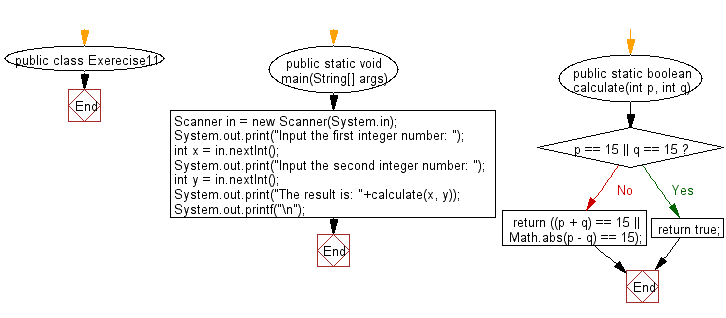﻿ Java Math Exercises: Accept two integers and return true if the either one is 15 or if their sum or difference is 15 - w3resource# Java Math Exercises: Accept two integers and return true if the either one is 15 or if their sum or difference is 15

## Java Math Exercises: Exercise-11 with Solution

Write a Java program to accept two integers and return true if the either one is 15 or if their sum or difference is 15.

Sample Solution:

Java Code:

``````import java.util.*;
public class Exerecise11 {
public static void main(String[] args)
{
Scanner in = new Scanner(System.in);
System.out.print("Input the first integer number: ");
int x = in.nextInt();
System.out.print("Input the second integer number: ");
int y = in.nextInt();
System.out.print("The result is: "+calculate(x, y));
System.out.printf("\n");
}

public static boolean calculate(int p, int q)
{
if(p == 15 || q == 15)
return true;
return ((p + q) == 15 || Math.abs(p - q) == 15);
}
}
``````

Sample Output:

```Input the first integer number: 15
Input the second integer number: 25
The result is: true
```

Flowchart:Java Code Editor:

What is the difficulty level of this exercise?

﻿

## Java: Tips of the Day

Parsing dates:

```import java.io.*;
import java.util.*;
import java.text.*;

String s = "2001/09/23 14:39";

SimpleDateFormat formatter = new SimpleDateFormat ("yyyy/MM/dd H:mm");
Date d = formatter.parse(s, new ParsePosition(0));
```